# Arithmetic group

(diff) ← Older revision | Latest revision (diff) | Newer revision → (diff)
A subgroupof a linear algebraic groupdefined over the fieldof rational numbers, that satisfies the following condition: There exists a faithful rational representation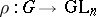defined over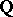(cf. Representation theory) such that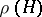is commensurable with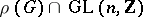, where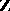is the ring of integers (two subgroupsandof a group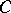are called commensurable if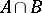is of finite index inand in). This condition is then also satisfied for any other faithful representation defined over. More generally, an arithmetic group is a subgroup of an algebraic group, defined over a global field, that is commensurable with the group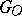of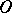-points of, whereis the ring of integers of. An arithmetic group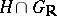is a discrete subgroup of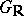.
If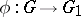is a-epimorphism of algebraic groups, then the image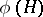of any arithmetic groupis an arithmetic group in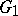. The name arithmetic group is sometimes also given to an abstract group that is isomorphic to an arithmetic subgroup of some algebraic group. Thus, ifis an algebraic number field, the group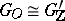, where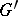is obtained fromby restricting the field of definition fromto, is called an arithmetic group. In the theory of Lie groups the name arithmetic subgroups is also given to images of arithmetic subgroups of the group of real points ofunder the factorization ofby compact normal subgroups.